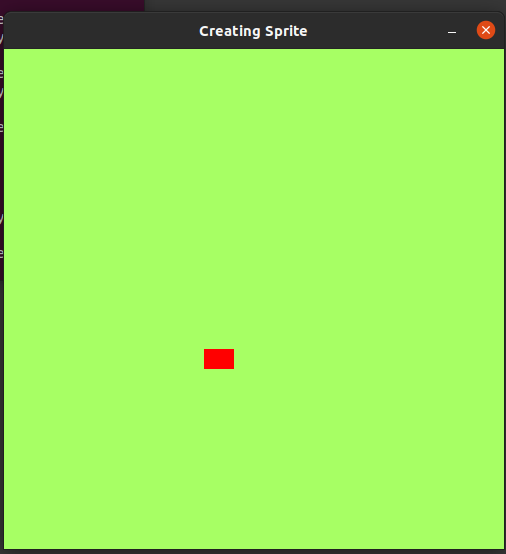Open In App
Related Articles
• Write an Interview Experience
• PyGame Tutorial

# Pygame – Creating Sprites

Sprites are objects, with different properties like height, width, color, etc., and methods like moving right, left, up and down, jump, etc. In this article, we are looking to create an object in which users can control that object and move it forward, backward, up, and down using arrow keys.

Let first look at our first-class i.e., the class in which our sprite is defined, we will call that class Sprite. This Sprite class defines its positions(x and y coordinates), dimension of an object, color, etc. First, we will be calling our __init__() method. It is called a constructor for a class.

Example: Creating Sprite class

## Python3

 `import` `pygame`` ` `# GLOBAL VARIABLES``COLOR ``=` `(``255``, ``100``, ``98``)``SURFACE_COLOR ``=` `(``167``, ``255``, ``100``)``WIDTH ``=` `500``HEIGHT ``=` `500`` ` `# Object class``class` `Sprite(pygame.sprite.Sprite):``    ``def` `__init__(``self``, color, height, width):``        ``super``().__init__()`` ` `        ``self``.image ``=` `pygame.Surface([width, height])``        ``self``.image.fill(SURFACE_COLOR)``        ``self``.image.set_colorkey(COLOR)`` ` `        ``pygame.draw.rect(``self``.image,``                         ``color,``                         ``pygame.Rect(``0``, ``0``, width, height))`` ` `        ``self``.rect ``=` `self``.image.get_rect()`

Now, that the class has been created, we can create objects from the class. It enables us to create as many objects as we need using the same class. Now we will create an object using our Class Sprite.

Syntax:

object = Sprite(RED,WIDTH,HEIGHT)

By default, the object will be on position (0,0) i.e., top-left of the screen. We can change the x and y properties of the object.

Syntax:

object.rect.x = value

object.rect.y = value

We can define n of sprites that we want to create, but for the purpose of understanding, let’s simplify. Here we have created a rectangle sprite of certain dimensions, on which we can perform different operations to perform on sprites like move forward, backward, jump, slow, accelerate, etc.

Example: Creating sprite

## Python3

 `import` `pygame``import` `random`` ` `# GLOBAL VARIABLES``COLOR ``=` `(``255``, ``100``, ``98``)``SURFACE_COLOR ``=` `(``167``, ``255``, ``100``)``WIDTH ``=` `500``HEIGHT ``=` `500`` ` `# Object class``class` `Sprite(pygame.sprite.Sprite):``    ``def` `__init__(``self``, color, height, width):``        ``super``().__init__()`` ` `        ``self``.image ``=` `pygame.Surface([width, height])``        ``self``.image.fill(SURFACE_COLOR)``        ``self``.image.set_colorkey(COLOR)`` ` `        ``pygame.draw.rect(``self``.image,color,pygame.Rect(``0``, ``0``, width, height))`` ` `        ``self``.rect ``=` `self``.image.get_rect()`` ` ` ` `pygame.init()`` ` `RED ``=` `(``255``, ``0``, ``0``)`` ` `size ``=` `(WIDTH, HEIGHT)``screen ``=` `pygame.display.set_mode(size)``pygame.display.set_caption(``"Creating Sprite"``)`` ` `all_sprites_list ``=` `pygame.sprite.Group()`` ` `object_ ``=` `Sprite(RED, ``20``, ``30``)``object_.rect.x ``=` `200``object_.rect.y ``=` `300`` ` `all_sprites_list.add(object_)`` ` `exit ``=` `True``clock ``=` `pygame.time.Clock()`` ` `while` `exit:``    ``for` `event ``in` `pygame.event.get():``        ``if` `event.``type` `=``=` `pygame.QUIT:``            ``exit ``=` `False`` ` `    ``all_sprites_list.update()``    ``screen.fill(SURFACE_COLOR)``    ``all_sprites_list.draw(screen)``    ``pygame.display.flip()``    ``clock.tick(``60``)`` ` `pygame.quit()`

Output: